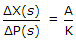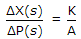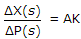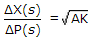# Electronics and Communication Engineering - Automatic Control Systems

### Exercise :: Automatic Control Systems - Section 6

1.

If a zero is added to a second order underdamped system

 A. the peak overshoot is increased B. the peak overshoot is decreased C. the peak overshoot remains unchanged D. either (a) or (b)

Explanation:

No answer description available for this question. Let us discuss.

2.

PID controlled system has

 A. P and I actions in forward path and D action in feedback path B. P and I actions in feedback path and actions in forward path C. All the three (i.e., P, I and D) actions in forward path D. All the three (i.e., P, I and D) actions in feedback path

Explanation:

No answer description available for this question. Let us discuss.

3.

In case of synchro error detector the electrical zero position of control transformer is obtained when angular displacement between rotors is

 A. zero B. 45° C. 90° D. 180°

Explanation:

No answer description available for this question. Let us discuss.

4.

If ΔP is pressure difference, A is area of each flat surface, K is stiffness and Δx is displacement of movable surface from reference of bellows, then

 A.B.C.D.Explanation:

No answer description available for this question. Let us discuss.

5.

For Bode plot of (1 + jωT) has

 A. slope of 20 dB/decade and phase angle + tan-1 (ωT) B. slope of - 20 dB/decade and phase angle + tan-1 (ωT) C. slope of 20 dB/decade and phase angle - tan-1 (ωT) D. slope of - 40 dB/decade and phase angle - tan-1(ωT)